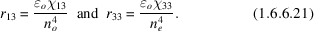International
Tables for
Crystallography
Volume D
Physical properties of crystals
Edited by A. Authier

International Tables for Crystallography (2006). Vol. D, ch. 1.6, pp. 172-173

## Section 1.6.6. Linear electro-optic effect

A. M. Glazera* and K. G. Coxb

aDepartment of Physics, University of Oxford, Parks Roads, Oxford OX1 3PU, England, and bDepartment of Earth Sciences, University of Oxford, Parks Roads, Oxford OX1 3PR, England
Correspondence e-mail:  glazer@physics.ox.ac.uk

### 1.6.6. Linear electro-optic effect

| top | pdf |

The linear electro-optic effect, given by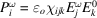, is conventionally expressed in terms of the change in dielectric impermeability caused by imposition of a static electric field on the crystal. Thus one may write the linear electro-optic effect as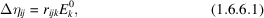where the coefficients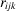form the so-called linear electro-optic tensor. These have identical symmetry with the piezoelectric tensor and so obey the same rules (see Table 1.6.6.1). Like the piezoelectric tensor, there is a maximum of 18 independent coefficients (triclinic case) (see Section 1.1.4.10.3). However, unlike in piezoelectricity, in using the Voigt contracted notation there are two major differences:

Typical values of linear electro-optic coefficients are around 10−12 mV−1.

#### 1.6.6.1. Primary and secondary effects

| top | pdf |

In considering the electro-optic effect, it is necessary to bear in mind that, in addition to the primary effect of changing the refractive index, the applied electric field may also cause a strain in the crystal via the converse piezoelectric effect, and this can then change the refractive index, as a secondary effect, through the elasto-optic effect. Both these effects, which are of comparable magnitude in practice, will occur if the crystal is free. However, if the crystal is mechanically clamped, it is not possible to induce any strain, and in this case therefore only the primary electro-optic effect is seen. In practice, the free and clamped behaviour can be investigated by measuring the linear birefringence when applying electric fields of varying frequencies. When the electric field is static or of low frequency, the effect is measured at constant stress, so that both primary and secondary effects are measured together. For electric fields at frequencies above the natural mechanical resonance of the crystal, the strains are very small, and in this case only the primary effect is measured.

#### 1.6.6.2. Example of LiNbO3

| top | pdf |

In order to understand how tensors can be used in calculating the optical changes induced by an applied electric field, it is instructive to take a particular example and work out the change in refractive index for a given electric field. LiNbO3 is the most widely used electro-optic material in industry and so this forms a useful example for calculation purposes. This material crystallizes in point group, for which the electro-optic tensor has the form (for the effect of symmetry, see Section 1.1.4.10) (withperpendicular to m)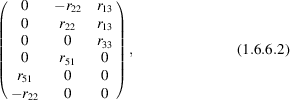with r13 = 9.6, r22 = 6.8, r33 = 30.9 and r51 = 32.5 × 10−12 mV−1, under the normal measuring conditions where the crystal is unclamped.

Calculation using dielectric impermeability tensor. Suppose, for example, a static electric field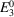is imposed along the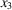axis. One can then write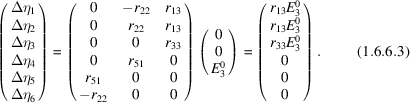Thus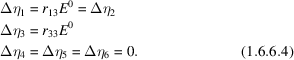Since the original indicatrix of LiNbO3 before application of the field is uniaxial,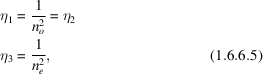and so differentiating, the following are obtained: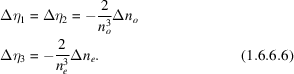Thus, the induced changes in refractive index are given by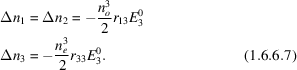It can be seen from this that the effect is simply to change the refractive indices by deforming the indicatrix, but maintain the uniaxial symmetry of the crystal. Note that if light is now propagated along, say,, the observed linear birefringence is given by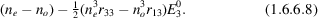If, on the other hand, the electric field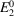is applied along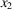, i.e. within the mirror plane, one finds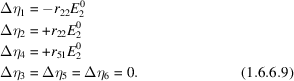Diagonalization of the matrix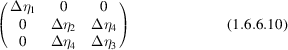containing these terms gives three eigenvalue solutions for the changes in dielectric impermeabilities: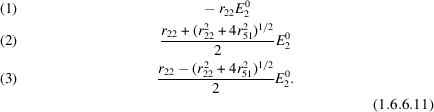On calculating the eigenfunctions, it is found that solution (1) lies along, thus representing a change in the value of the indicatrix axis in this direction. Solutions (2) and (3) give the other two axes of the indicatrix: these are different in length, but mutually perpendicular, and lie in the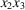plane. Thus a biaxial indicatrix is formed with one refractive index fixed alongand the other two in the plane perpendicular. The effect of having the electric field imposed within the mirror plane is thus to remove the threefold axis in point group 3m and to form the point subgroup m (Fig. 1.6.6.1).Figure 1.6.6.1 | top | pdf |(a) Symmetry elements of point group 3m. (b) Symmetry elements after field applied along. (c) Effect on circular section of uniaxial indicatrix.

Relationship between linear electro-optic coefficientsand the susceptibility tensor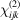. It is instructive to repeat the above calculation using the normal susceptibility tensor and equation (1.6.3.14). Consider, again, a static electric field alongand light propagating along. As before, the only coefficients that need to be considered with the static field alongare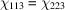and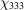. Equation (1.6.3.14)can then be written as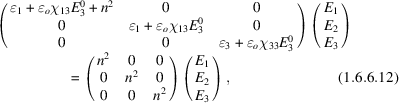where for simplicity the Voigt notation has been used. The first line of the matrix equation gives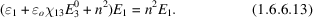Since only a transverse electric field is relevant for an optical wave (plasma waves are not considered here), it can be assumed that the longitudinal field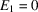. The remaining two equations can be solved by forming the determinantal equation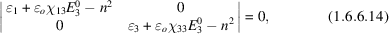which leads to the results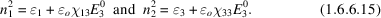Thus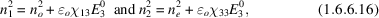and so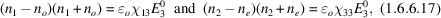and since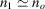and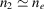,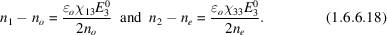Subtracting these two results, the induced birefringence is found: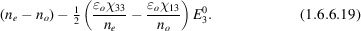Comparing with the equation (1.6.6.8)calculated for the linear electro-optic coefficients,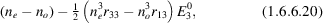one finds the following relationships between the linear electro-optic coefficients and the susceptibilities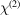: